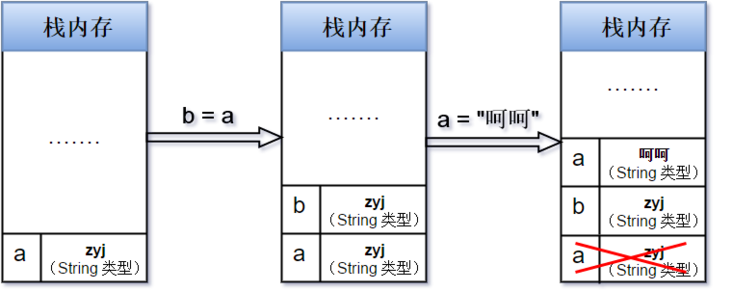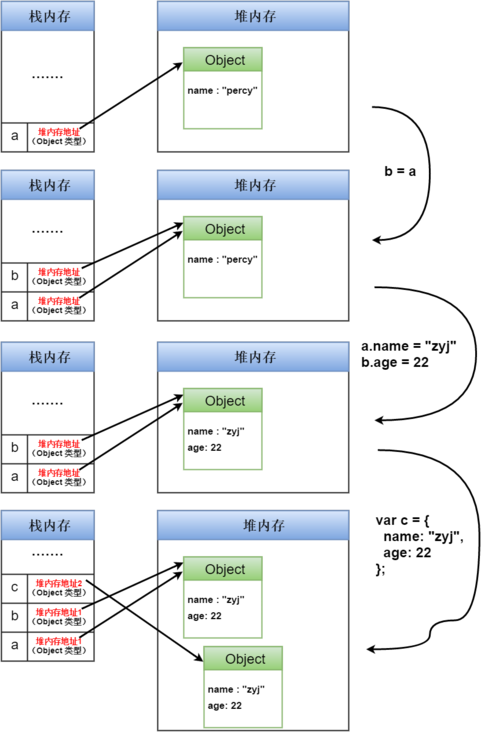# JavaScript 深入了解基本类型和引用类型的值

epeppanda

0收藏

ES6 引入了一种新的原始数据类型 Symbol，表示独一无二的值。它是 JavaScript 语言的第七种数据类型，前六种是：Undefined、Null、布尔值（Boolean）、字符串（String）、数值（Number）、对象（Object）。

JavaScript 中有 6 种基本数据类型：Undefined、Null、Boolean、Number、String、Symbol (new in ES 6) ！

``````var str = "123hello321";
str.toUpperCase();     // 123HELLO321
console.log(str);      // 123hello321``````

``````var a = 1;
var b = true;
console.log(a == b);    // true
console.log(a === b);   // false``````

== : 只进行值的比较
=== : 不仅进行值得比较，还要进行数据类型的比较

``````var a,b;
a = "zyj";
b = a;
console.log(a);   // zyj
console.log(b);   // zyj
a = "呵呵";       // 改变 a 的值，并不影响 b 的值
console.log(a);   // 呵呵
console.log(b);   // zyj````````````var obj = {name:"zyj"};   // 创建一个对象
obj.name = "percy";       // 改变 name 属性的值
obj.age = 21;             // 添加 age 属性
obj.giveMeAll = function(){
return this.name + " : " + this.age;
};                        // 添加 giveMeAll 方法
obj.giveMeAll();``````

``````var obj1 = {};    // 新建一个空对象 obj1
var obj2 = {};    // 新建一个空对象 obj2
console.log(obj1 == obj2);    // false
console.log(obj1 === obj2);   // false``````

``````var a = {name:"percy"};
var b;
b = a;
a.name = "zyj";
console.log(b.name);    // zyj
b.age = 22;
console.log(a.age);     // 22
var c = {
name: "zyj",
age: 22
};``````

• 栈内存中保存了变量标识符和指向堆内存中该对象的指针
• 堆内存中保存了对象的内容• typeof：经常用来检测一个变量是不是最基本的数据类型
``````var a;
typeof a;    // undefined

a = null;
typeof a;    // object

a = true;
typeof a;    // boolean

a = 666;
typeof a;    // number

a = "hello";
typeof a;    // string

a = Symbol();
typeof a;    // symbol

a = function(){}
typeof a;    // function

a = [];
typeof a;    // object
a = {};
typeof a;    // object
a = /aaa/g;
typeof a;    // object   ​``````

instanceof：用来判断某个构造函数的 prototype 属性所指向的对象是否存在于另外一个要检测对象的原型链上

``````({}) instanceof Object              // true
([]) instanceof Array               // true
(/aa/g) instanceof RegExp           // true
(function(){}) instanceof Function  // true``````